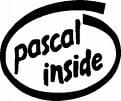0
Thanks

A few words of thanks would be greatly appreciated.

# Pascal Language - Shift array of elements (Rotation)-RecursionHere is a recursive procedure which allows to shift all the elements of an array to the right from the position p.

```Procedure Shift (Var t : Tab; p, n : integer);
Begin
If p <= n Then
Begin
Decaler (t, p + 1, n);
t[p + 1] := t[p];
End;
End;
```

Thanks to Zouari Lazhar for this tip.

0
Thanks

A few words of thanks would be greatly appreciated.